# Worksheet 1 - Measurement Notes | EduRev

## Class 1 : Worksheet 1 - Measurement Notes | EduRev

The document Worksheet 1 - Measurement Notes | EduRev is a part of the Class 1 Course Mathematics (Maths) for Class 1.
All you need of Class 1 at this link: Class 1

Look at the pictures in the boxes. Answer the question by circling the correct picture
Q.1. Which is longer?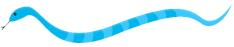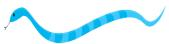Ans.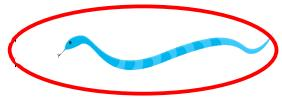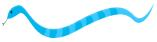Q.2. Which is shorter?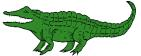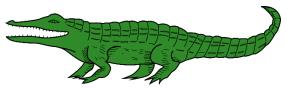Ans.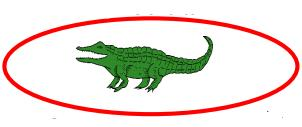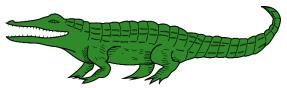Q.3. Which is longer?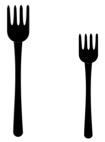Ans.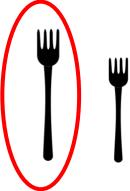Q.4. Which is shorter?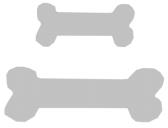Ans.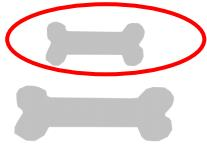Q.5. Which is longer?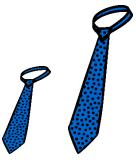Ans.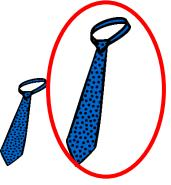Q.6. Which is shorter?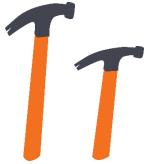Ans.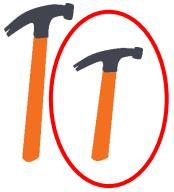Q.7. Which is taller?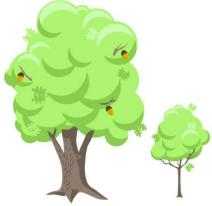Ans.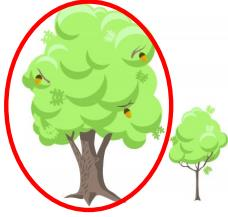Q.8. Which is shorter?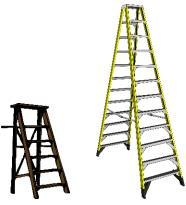Ans.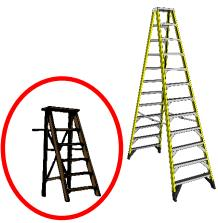Q.9. Which is taller?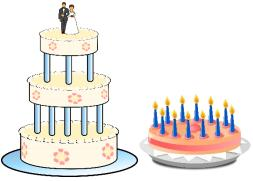Ans.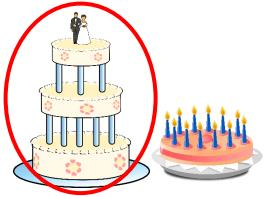Q.10. Which is shorter?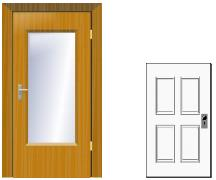Ans.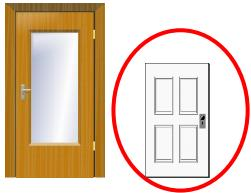Q.11. Which is taller?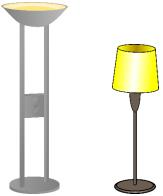Ans.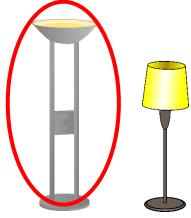Q.12. Which is shorter?Ans.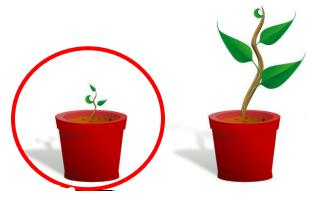Q.13. Order the three objects from the shortest to the longest. Write “1” under the shortest object and “3” under the longest object.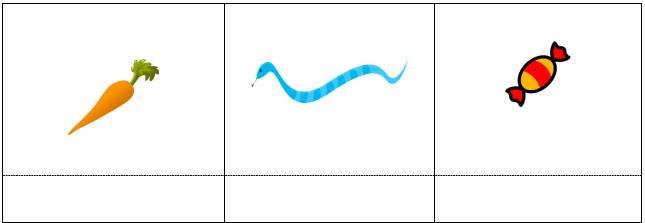Ans.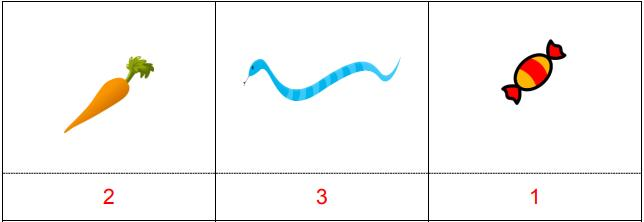Q.14. Order the three objects from the shortest to the longest. Write “1” under the shortest object and “3” under the longest object.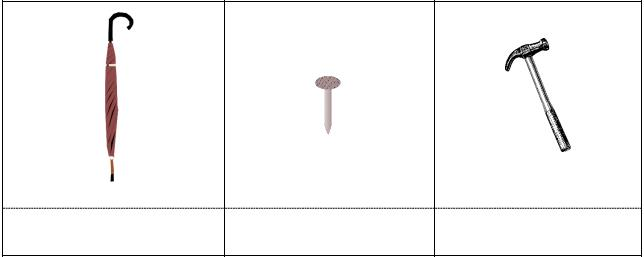Ans.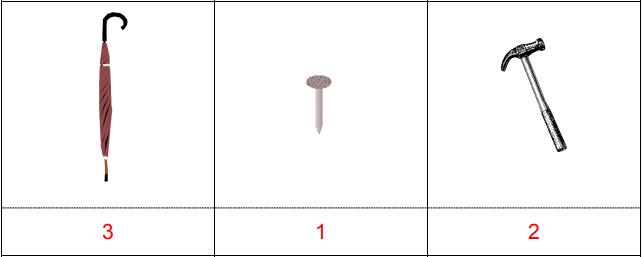Q.15. Order the three objects from the shortest to the longest. Write “1” under the shortest object and “3” under the longest object.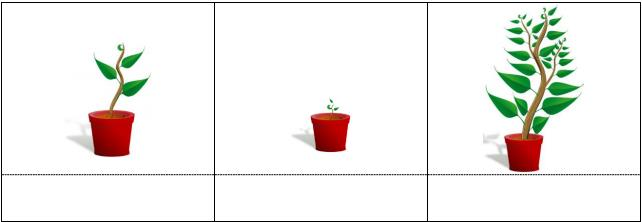Ans.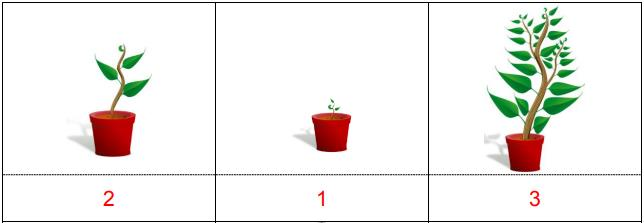Q.16. Order the three objects from the shortest to the longest. Write “1” under the shortest object and “3” under the longest object.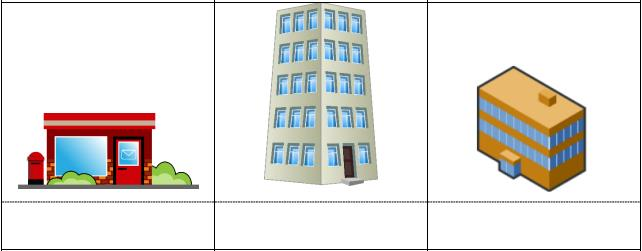Ans.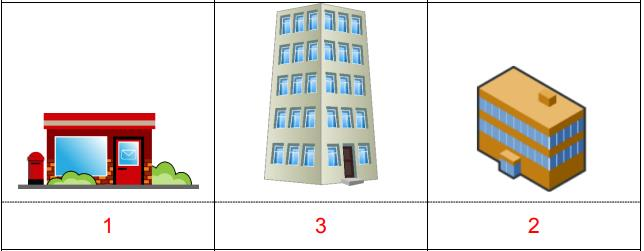Q.17. Order the three objects from the shortest to the longest. Write “1” under the shortest object and “3” under the longest object.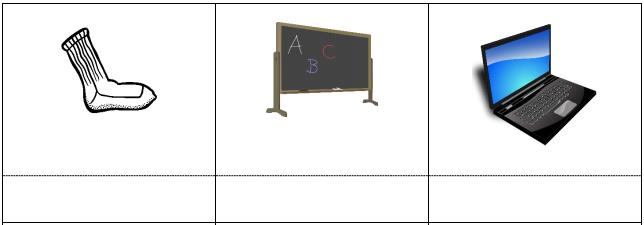Ans.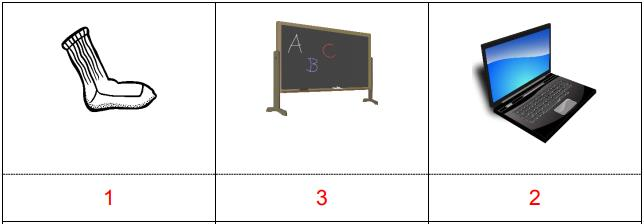Q.18. Order the three objects from the shortest to the longest. Write “1” under the shortest object and “3” under the longest object.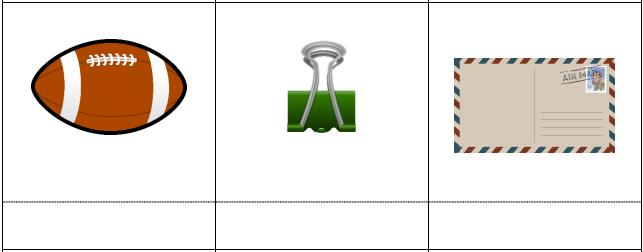Ans.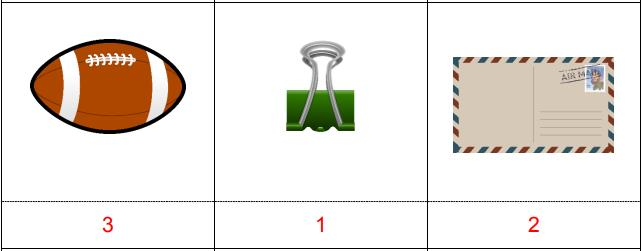Q.19. Use a penny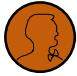to measure each picture below.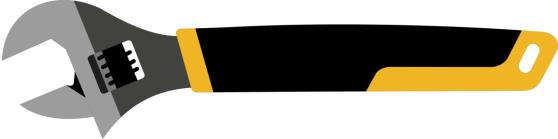Length: ______ pennies
Ans. Wrench: Length = 8 pennies

Q.20. Measure the following objects using the ruler provided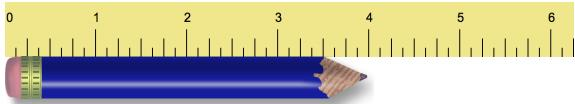Length: __________ inches
Ans. Pencil: 4 inches

Q.21. Measure the following objects using the ruler provided.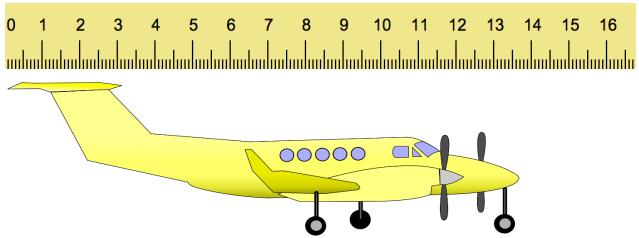Length: __________ centimeters
Ans. Plane: 14 centimeters

Q.22. Using the ruler, measure the following crayons and circle the longest crayon.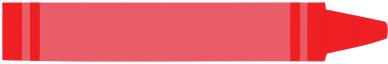__________ inches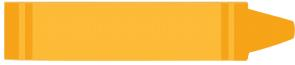__________ inches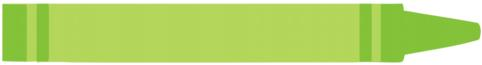__________ inches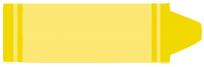__________ inches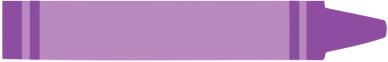__________ inches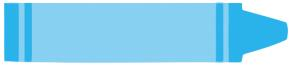__________ inches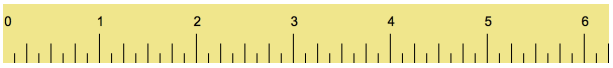Ans.
Red: 4 inches
Orange: 3 inches
Green: 5 inches
Yellow: 2 inches
Purple: 4 inches
Blue: 3 inches
Longest crayon – green

Q.23. Using the ruler, measure the following trains and circle the longest train.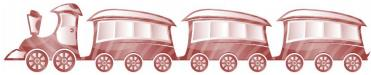_____ centimeters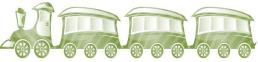_____ centimeters_____ centimeters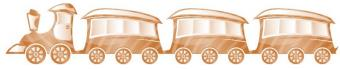_____ centimeters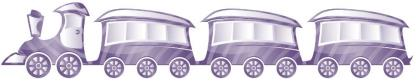_____ centimeters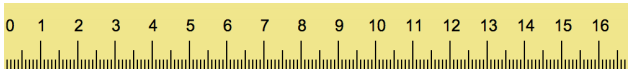Ans.
Red: 10 centimeters
Green: 7 centimeters
Blue: 12 centimeters
Orange: 9 centimeters
Purple: 11 centimeters
Longest train – blue

Q.24. Measure the following objects and circle the longer object.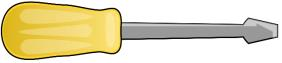Length: __________ inches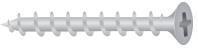Length: __________ inches
Ans.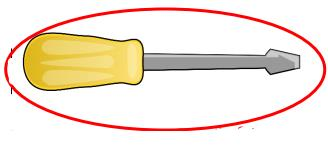Length: 3 inches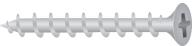Length: 2 inches

Q.25. Measure the following objects and circle the shorter object.Length: __________ centimetersLength: __________ centimeters
Ans.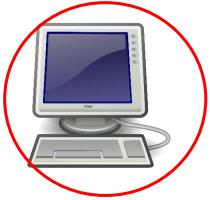Length: 4 centimetersLength: 8 centimeters

Offer running on EduRev: Apply code STAYHOME200 to get INR 200 off on our premium plan EduRev Infinity!

## Mathematics (Maths) for Class 1

59 videos|43 docs|25 tests

,

,

,

,

,

,

,

,

,

,

,

,

,

,

,

,

,

,

,

,

,

;